#### Howdy, Stranger!

We are about to switch to a new forum software. Until then we have removed the registration on this forum.

# Descending curve function?

edited September 2017

My math skills have faded with my hair color, I'm afraid. I need a function that produces a downward curve. For example, I will send it integers from 255-0. This is for a fading effect, the numbers are transparency values. I want it to fade quickly at first then to fade more slowly as it gets closer to zero. Ideally I'd like some control over the steepness (or "curviness") of the curve.

Suggestions? :)

--Darin

Tagged:

• ``````float[] lookup = new float;

void setup(){
size(256,256);
for( int i = 0; i < lookup.length; i++ ){
lookup[i] = 255 - map(i*i, 0, 256*256, 0, 256);
}
}

void draw(){
background( (mouseX >= 0 && mouseX < lookup.length) ? (lookup[mouseX]) : (0) );
stroke(255,0,0);
for( int i = 0; i < lookup.length; i++ ){
point( i, lookup[i] );
}

}
``````
• edited September 2017 Answer ✓

An alternative approach using a class to generate next opacity value: \$-)
Online version: https://OpenProcessing.org/sketch/450448

``````/**
* AlphaStepGen (v1.0)
* GoToLoop (2017/Sep/20)
*
* Forum.Processing.org/two/discussion/24193/descending-curve-function#Item_2
* OpenProcessing.org/sketch/450448
*/

static final boolean JS = 1/2 == 1/2.;
final AlphaStep opaque = new AlphaStep();

void setup() {
size(800, 600);
smooth(3);
frameRate(5);

colorMode(RGB);
ellipseMode(CENTER);

strokeWeight(2.5);
stroke(0);

println(opaque.steps);
}

void draw() {
background(-1);
fill(255, 0, 0, opaque.nextAlpha());
ellipse(width>>1, height>>1, width>>1, height>>1);

if (JS)  return;

String info = "Frame: " + frameCount + "  -  Alpha: " + opaque.toString();
getSurface().setTitle(info);
}

class AlphaStep {
static final int STEP = 5;
float opacity = 255;
final float[] steps = new float[1 + (int)opacity];

AlphaStep() {
for (int opac = (int)opacity, i = steps.length; i-- > 0;
steps[opac - i] = STEP * norm(i, opac, 0));
}

color nextAlpha() {
return round(opacity -= steps[round(opacity)]);
}

String toString() {
return nf(opaque.opacity, 0, 2);
}
}
``````
• edited September 2017 Answer ✓

@darinb --

Non-linear curve-based interpolation along a single dimension based on control values is already built-in to Processing in curvePoint() and bezierPoint().

You can use it like this:

``````value = bezierPoint(min, in, max-out, max, t)
``````

So, if I want values 0-255 and I'm exerting 300 worth of control to the 'out' end of the curve (making it more shallow), then:

``````transparency = bezierPoint(0, 0, 255-300, 255, t);
``````

...where `t` is a time-based value 0-1.0 and the return is 0-255 along that time curve.

I find curve-based interpolation easier to use if I wrap it in a helper function that makes the arguments easier to understand:

``````float cerp(float min, float max, float in, float out, float t){
return bezierPoint(min, in, max-out, max, t);
}
``````

So:

``````transparency = cerp(0, 255, 0, 300, t);
``````

To see it in action, check out this demo sketch:

``````/**
* cerp -- curve interpolation
* 2017-09-21 Jeremy Douglass Processing 3.3.6
*
* The built-in functions bezierDetail or curveDetail can be used
* for curve-based interpolation -- such as time/transparency fades
* which are not linear, but start fast / end slow etc.
* This sketch demonstrates using bezierDetail as a 'cerp' function.
*/

float transparency;
float t;
float duration;
float ctrl;

void setup() {
t = 0;
duration = 3000; // cycle every n milliseconds
ctrl = 300;
}

float cerp(float min, float max, float in, float out, float t){
return bezierPoint(min, in, max-out, max, t);
}

void draw() {
background(255);

// set timer to a value 0.0-1.0
// based on current time and cycle duration
t = (millis()/duration) % 1.0;

transparency = cerp(0, 255, 0, 0, t);
fill(ctrl,0,0,transparency);
rect(10,10,35,35);

transparency = cerp(0, 255, 0, ctrl, t);
fill(255,0,0,transparency);
rect(10,55,35,35);

transparency = cerp(0, 255, ctrl, 0, t);
fill(255,0,0,transparency);
rect(55,10,35,35);

transparency = cerp(0, 255, ctrl, ctrl, t);
fill(255,0,0,transparency);
rect(55,55,35,35);

fill(0);
text("linear", 10, 10);
text("out", 10, 55);
text("in", 55, 10);
text("in/out", 55, 55);
text(t, 10, 100);
}
``````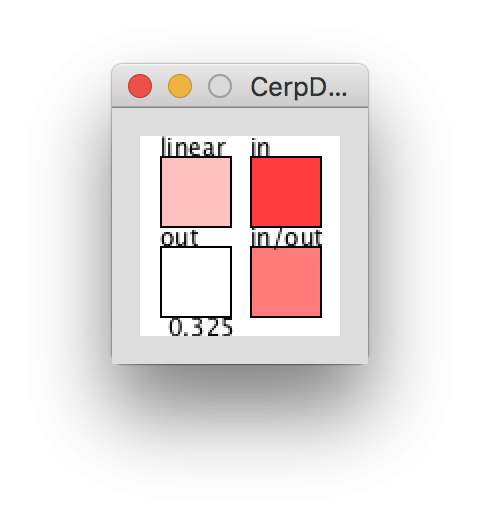• edited September 2017 Answer ✓

If you are interested in understanding how the `bezierPoint()` 1D process relates to 2D Bezier curves, watch this sketch, then try pressing 'i' / 'o' to turn on and off the in and out control steppers:

``````/**
* BezierControlDemo
* 2017-09-21 Jeremy Douglass Processing 3.3.6
*
* Understand how changing in the x values of bezier() control points produces a response
* in the x return values of bezierPoint(). Useful for understanding cerp (curve-based interpolation).
* Press 'i' / 'o' to turn on and off in and out control steppers.
*/

float size = 255;
float margin = 10;
float controlIn = 0;
float controlOut = 0;
float t;
float x;
float y;

boolean stepIn;
boolean stepOut;

void settings() {
size(int(size+2*margin), int(size+2*margin));
}
void setup() {
noFill();
stepIn = true;
stepOut = true;
}
void draw() {
background(192);
translate(margin, margin);

// draw grid
stroke(128);
for (float i=0; i<=size; i+=size/10) {
line(0,i,size,i);
line(i,0,i,size);
}

// draw control points
stroke(255, 102, 0);
line(controlIn, 0, 0, 0);
ellipse(controlIn, 0, margin, margin);
line(size-controlOut, size, size, size);
ellipse(size-controlOut, size, margin, margin);

// draw curve
stroke(0, 0, 0);
bezier(0, 0, controlIn, 0, size-controlOut, size, size, size);

// draw points on the curve
fill(255);
for (float i=0; i<1; i+=0.1) {
float rx = bezierPoint(0, controlIn, size-controlOut, size, i);
float ry = bezierPoint(0, 0, size, size, i);
stroke(0);
ellipse(rx, ry, 5, 5);
}
noFill();

// draw a time-based point moving on the curve
x = bezierPoint(0, controlIn, size-controlOut, size, t);
y = bezierPoint(0, 0, size, size, t);
ellipse(x, y, margin, margin);

// update the time step
t += 0.01;

// reset the time step and update new control points
if (t>1) {
t = 0;
if (stepIn){
controlIn += size/10;
}
if (stepOut){
controlOut += size/10;
}
if (controlIn > size || controlOut > size) {
controlIn = 0;
controlOut = 0;
}
}
}

// turn control point stepping off and on
void keyPressed(){
if(key == 'i'){
stepIn = !stepIn;
}
if(key == 'o'){
stepOut = !stepOut;
}
}
``````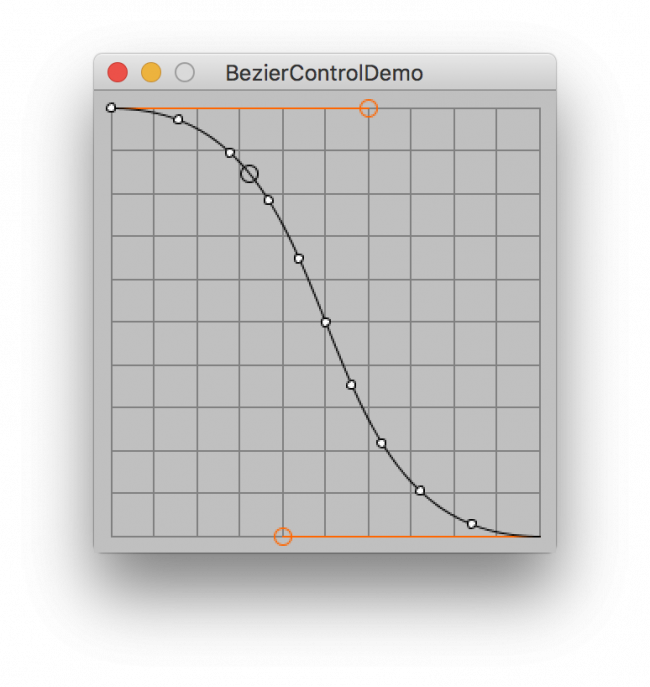• Wow--what a great forum. Not only is my question answered but I've learned about the map function, an object version (still trying to get a handle on object code), and a mini-course on bezier curves in Processing and how to use them. Really outstanding. You should add this to the documentation.....

Really, thanks to everyone. I'm so glad I found Processing (after a bit of a struggle with pyGame) and so glad this forum is here.

--Darin

• edited September 2017

I also had some fun trying to understand bezier creation...

try running this

``````PVector t1, t2, t3;
float s ;
PVector p1, p2, p3;

ArrayList<PVector> points = new ArrayList<PVector>();

void setup() {
size(600, 600, P2D);
p1 = new PVector(0, 0);
p2 = new PVector(-120, 200);
p3 = new PVector(100, 230);
smooth(8);
strokeWeight(1.7);
}

void draw() {
background(255);
translate(width/2, height/2);
noStroke();
fill(200, 250, 250);
ellipse(p1.x, p1.y, 10, 10);
ellipse(p2.x, p2.y, 10, 10);
ellipse(p3.x, p3.y, 10, 10);

if (s<=1) {
s+=0.002;
t1 = PVector.lerp(p1, p2, s);
t2 = PVector.lerp(p2, p3, s);
t3 = PVector.lerp(t1, t2, s);
} else {
t1.set(0f, 0f);
t2.set(0f, 0f);
s= 0f;
points.clear();
p3 = PVector.random2D();
p3.mult(random(100, 280));

p2 = PVector.random2D();
p2.mult(random(100, 280));
}

noFill();
strokeWeight(1.7);
stroke(0, 0, 200);

for (PVector p : points) {
point(p.x, p.y);//, 4, 4);
}

strokeWeight(1);
stroke(200);
line(p1.x, p1.y, p2.x, p2.y);
line(p2.x, p2.y, p3.x, p3.y);

stroke(140, 150, 150);
line(t1.x, t1.y, t2.x, t2.y);

noStroke();
fill(200, 50, 50);
ellipse(t1.x, t1.y, 4, 4);
ellipse(t2.x, t2.y, 4, 4);
ellipse(t3.x, t3.y, 6, 6);
}
``````
• That's a very cool Bezier demo, @_vk !

One thing to keep in mind about the original question about time interpolation is that it is 1D, not 2D -- you put the time and some parameters into a single `bezierPoint()` call, not two calls, and the value you get back could be considered the x component only of a complex curve -- it is a non-linear value distribution that uses the x components of the control "points", here just control values.

• Thanks @jeremydouglass. Good point.

• O.K., I'm still trying to understand this--thought I did but I'm getting unexpected results. I put together a slightly modified version of jeremydouglass' code (his first example)--but I'm getting negative numbers initially coming out of the cerp function--which doesn't make sense to me.

The results of the cerp function start out slightly negative then turn around an go in the correct (positive) direction.

Here is what I have to test--the first line of the output in the raw result of the cerp function.

``````float transparency;
float transparencyReductionfactor;
float t;
float duration;
float ctrl;

void setup() {
t = 0;
duration = 3000; // cycle every n milliseconds
ctrl = 300;
}

float cerp(float min, float max, float in, float out, float t){
return bezierPoint(min, in, max-out, max, t);
}

void draw() {
background(255);

// set timer to a value 0.0-1.0
// based on current time and cycle duration
t = (millis()/duration) % 1.0;

transparencyReductionfactor = cerp(0, 255, 0, ctrl, t);
transparency = 255 - transparencyReductionfactor;
fill(0);
text(str(transparencyReductionfactor), 10, 10);
text(str(transparency), 10, 30);
text (str(t), 10, 50);
}
``````

My guess here is that the third parameter in the cerp function might be not what I want? As I understand it (perhaps dimly), the third and fourth parameter are specifying a point, forcing the curve to go through that point on its way from the begin point and end point (thus the Bezier curve). So is having that point at zero on the x-axis forcing the beginning of the curve into negative territory?

Or....? :)

--Darin

• Your max value is 255. Your out control is 300. That's probably not what you want -- if you don't want your values to go negative, the max out control you want is 255.

See this illustration from the demo sketch I shared above. Out (the orange line, 350) is greater than the range of min-max values (0-255), which causes the curve to become concave like a C.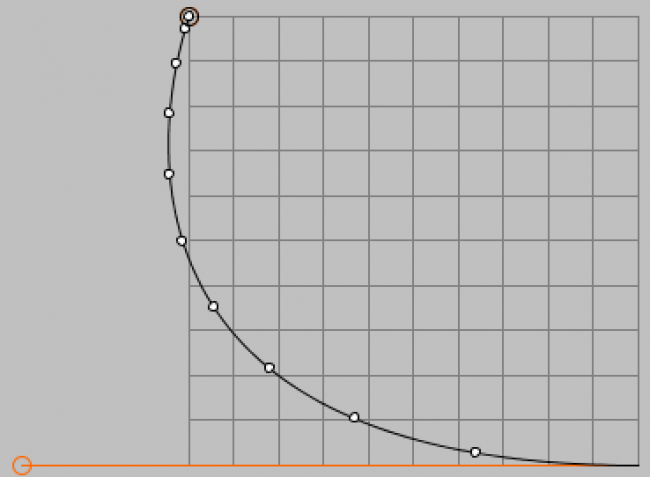Compare out control set = 255.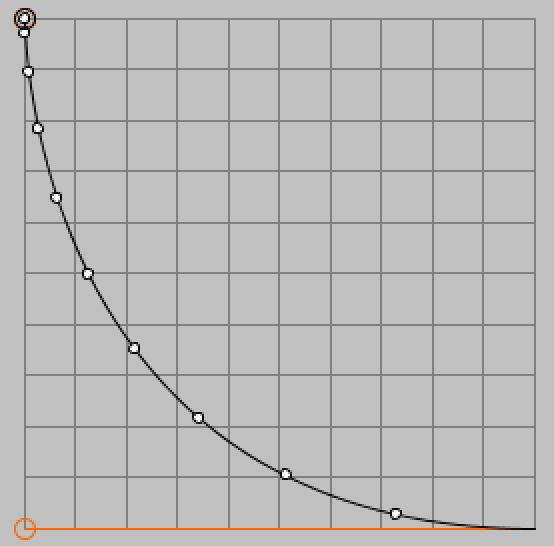• And of course, this is with a small out control value, = 128.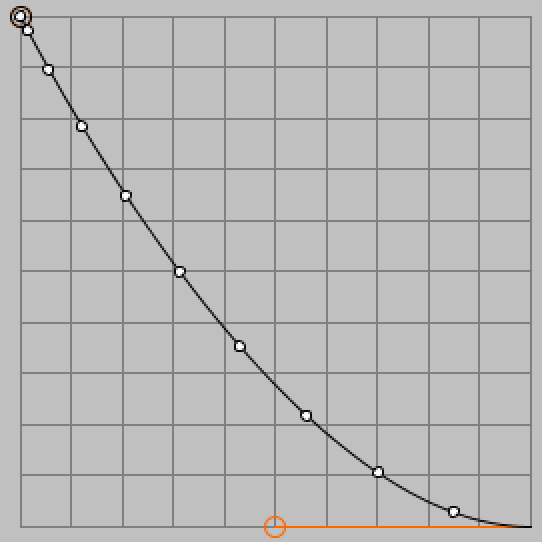• Got it working and I understand it now. Thanks!

--Darin